# Initialize Anomaly Detection Model (GMM-CV) VI

LabVIEW 2018 Analytics and Machine Learning Toolkit Help

Edition Date: July 2018

Part Number: 377059B-01

»View Product InfoDownload Help (Windows Only)

Owning Palette: Anomaly Detection VIs

Requires: Analytics and Machine Learning Toolkit

Initializes the hyperparameters of the Gaussian mixture model (GMM) algorithm.

Use the Train Anomaly Detection Model VI to train the GMM baseline model with training data. Use the Deploy Anomaly Detection Model VI to deploy the GMM baseline model and calculate the confidence value (CV) of the data for deployment. The Deploy Anomaly Detection Model VI calculates the CV by computing the overlap between the GMM baseline model and the GMM model. The Deploy Anomaly Detection Model VI uses health index to return the CV.

You can initialize a GMM model for batch training with this VI when you have a large training data set. To improve the machine learning model quality and the predictive performance, NI recommends that you shuffle the data set before training so each batch has similar distribution.

Use the pull-down menu to select an instance of this VI.

 Select an instance One ShotBatch

## One Shot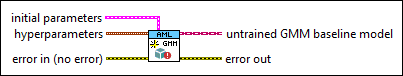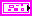initial parameters specifies the initial parameters to train the GMM baseline model.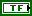auto initialization specifies whether this VI automatically calculates the values in initial parameters using K-Means algorithm. The default is TRUE, which specifies that this VI automatically sets the values in initial parameters using K-Means algorithm.

If you do not specify values for the arrays in initial parameters and auto initialization is FALSE, this VI randomly calculates the values in initial parameters.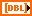initial mean values specifies the initial mean values of all Gaussian distributions in the GMM baseline model.initial covariance values specifies the initial covariance values of all Gaussian distributions in the GMM baseline model.initial covariance matrix specifies the initial covariance matrix of each Gaussian distribution in the GMM baseline model.initial weights specifies the initial weights of all Gaussian distributions in the GMM baseline model.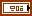hyperparameters specifies the hyperparameters to train the GMM baseline model.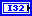number of clusters specifies the number of Gaussian distributions in the GMM baseline model. The default is 3.regulator specifies the value that this VI adds to the covariance matrix to prevent the covariance matrix from converging to 0. The default is 0.000001.tolerance specifies the tolerance for the stopping criteria. The default is 0.0001. The model fitting stops if the change of maximum likelihood between two successive optimization iterations is lower than tolerance.max iteration specifies the maximum number of optimization iterations for the stopping criteria. The default is 100. The model fitting stops if the number of optimization iterations reaches max iteration.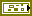error in describes error conditions that occur before this node runs. This input provides standard error in functionality.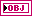untrained GMM baseline model returns the initialized GMM baseline model for training.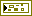error out contains error information. This output provides standard error out functionality.

## Batch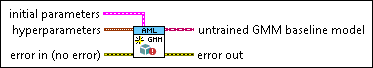initial parameters specifies the initial parameters to train the GMM baseline model.auto initialization specifies whether this VI automatically calculates the values in initial parameters using K-Means algorithm. The default is TRUE, which specifies that this VI automatically sets the values in initial parameters using K-Means algorithm.

If you do not specify values for the arrays in initial parameters and auto initialization is FALSE, this VI randomly calculates the values in initial parameters.initial mean values specifies the initial mean values of all Gaussian distributions in the GMM baseline model.initial covariance values specifies the initial covariance values of all Gaussian distributions in the GMM baseline model.initial covariance matrix specifies the initial covariance matrix of each Gaussian distribution in the GMM baseline model.initial weights specifies the initial weights of all Gaussian distributions in the GMM baseline model.hyperparameters specifies the hyperparameters to train the GMM baseline model.number of clusters specifies the number of Gaussian distributions in the GMM baseline model. The default is 3. For the first batch data, number of clusters must be less than or equal to the number of samples.regulator specifies the value that this VI adds to the covariance matrix to prevent the covariance matrix from converging to 0. The default is 0.000001.error in describes error conditions that occur before this node runs. This input provides standard error in functionality.untrained GMM baseline model returns the initialized GMM baseline model for training.error out contains error information. This output provides standard error out functionality.

## Initialize Anomaly Detection Model (GMM-CV) Details

The following equation defines the GMM model:where K is the number of Gaussian mixture components. Each Gaussian mixture component represents a Gaussian distribution. μi and Σi are the mean value and the covariance value of the ith Gaussian mixture component αi is the weight of the ith Gaussian mixture component

## Examples

Refer to the following VIs for examples of using the Initialize Anomaly Detection Model (GMM-CV) VI:

• Anomaly Detection (Training) VI: labview\examples\AML\Anomaly Detection
• Anomaly Detection (Training) (Batch) VI: labview\examples\AML\Anomaly Detection

Not Helpful